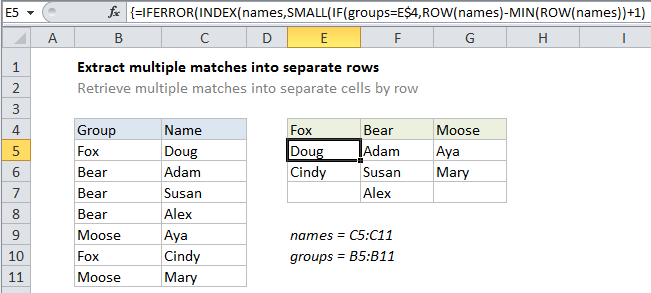## Excel Office

Excel How Tos, Tutorials, Tips & Tricks, Shortcuts

# Extract multiple matches into separate rows in Excel

This tutorial shows how to calculate Extract multiple matches into separate rows in Excel using the example below;## Explanation

To extract multiple matches to separate cells, in separate rows, you can use an array formula based on INDEX and SMALL. In the example shown, the formula in E5 is:

```{=IFERROR(INDEX(names,SMALL
(IF(groups=E\$4,ROW(names)-MIN(ROW
(names))+1),ROWS(\$E\$5:E5))),"")}```

This is an array formula and must be entered with Control + Shift + Enter.

After you enter the formula in the first cell, drag it down and across to fill in the other cells.

Also See:   Categorize text with keywords in Excel

### How this formula works

Note: this formula uses two named ranges: “names” refers to C4:C11, and “groups” refers to B4:B11. These names are defined in the screen shot above as well.

The gist of this formula is this: we are using the SMALL function to get a row number that corresponds to an “nth match”. Once we have the row number, we simply pass it into the INDEX function function, which returns the value at that row.

The trick is that SMALL is working with an array that is dynamically constructed by IF in this bit:

`IF(groups=E\$4,ROW(names)-MIN(ROW(names))+1)`

This snippet tests the named range “groups” for the value in E4. If found, it returns a “normalized” row number from an array of row numbers created with this part of the formula:

`ROW(names)-MIN(ROW(names))+1`

The result is an array that contains row numbers where there is a match, and FALSE where not. The array looks something like this:

Also See:   List sheet names with formula in Excel

{1;FALSE;FALSE;FALSE;FALSE;6;FALSE}

This array goes into SMALL. The k value for SMALL (nth) comes from an expanding range:

`ROWS(\$E\$5:E5)`

When copied down the result table, the range expands, causing k (nth) to increment. The SMALL function returns each matching row number, which is supplied to the INDEX function as row_num, with the named range “names” as the array.

Also See:   SMALL function: Description, Usage, Syntax, Examples and Explanation

### Handling errors

When ROWS returns a value for k that does not exist, SMALL throws a #NUM error. This happens after all matches have occurred. To suppress the error, we use IFERROR to catch the error and return an empty string (“”).